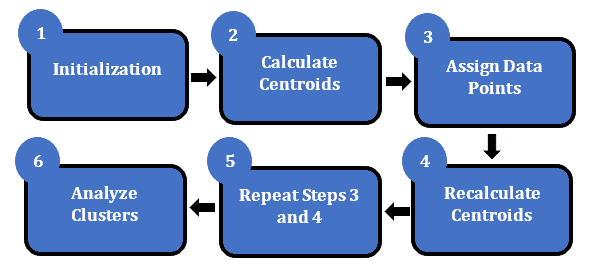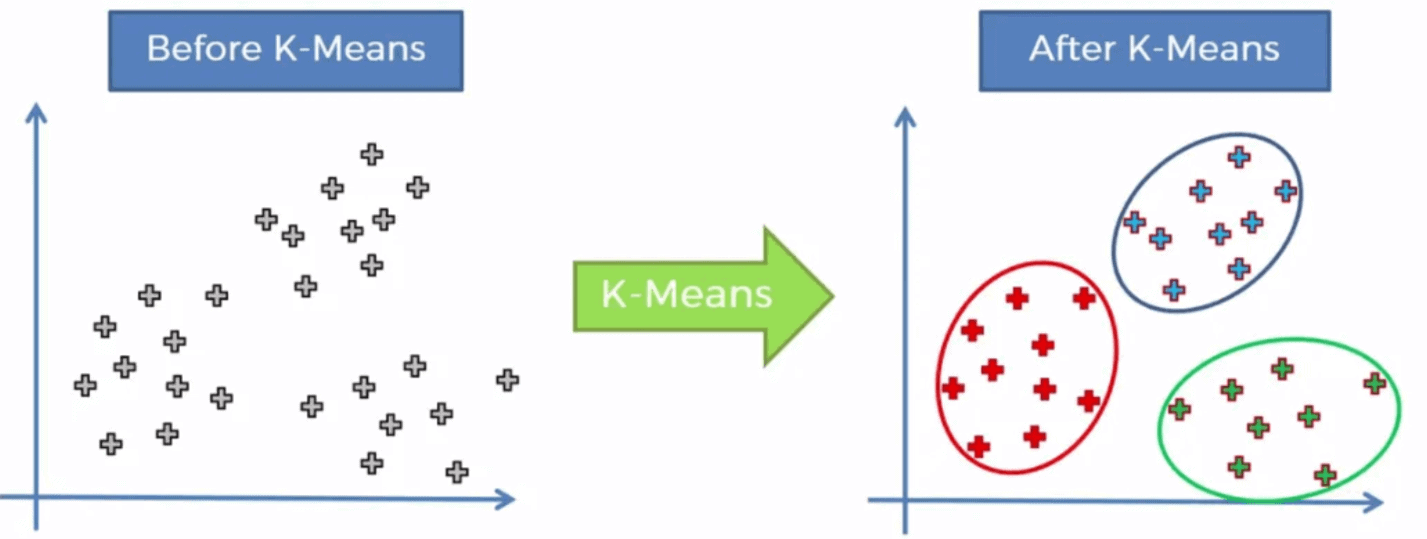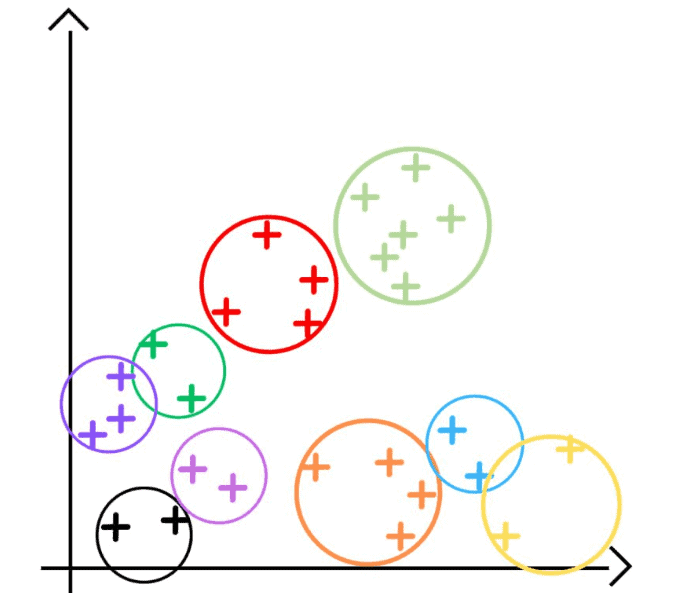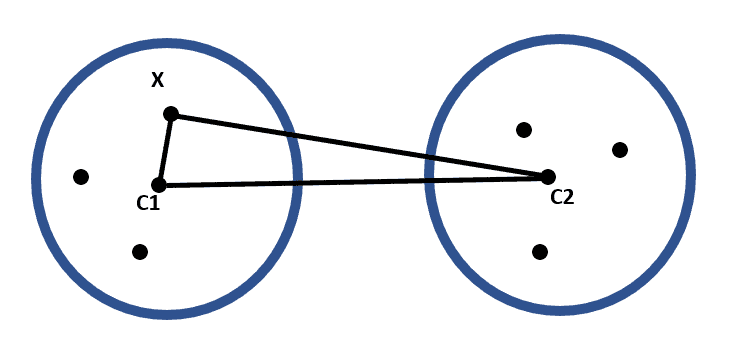## 1. Introduction

In this tutorial, we’ll talk about the K-means algorithm. We’ll examine its limitations and explore alternative approaches that address these drawbacks.

## 2. K-Means Algorithm

The K-means algorithm is a machine-learning algorithm for clustering. This type of learning is unsupervised. We use K-Means to group similar data items based on their differences and similarities. It has many applications, including anomaly detection, customer segmentation, and picture segmentation.It seeks to reduce the distances between each data item and its assigned centroid. This is an iterative process, starting with random assignments and gradually improving the clusters by adjusting the centroids based on the data points assigned to each cluster.

For example:## 3. Drawbacks of the K-Means Algorithm

While the K-means algorithm is widely used and has many advantages, it also has some drawbacks that should be considered.

### 3.1. Sensitivity to Initial Conditions

K-means is sensitive to initial conditions. The algorithm randomly initializes the cluster centroids at the beginning, and the final clustering results can vary depending on these initial positions.

Different initializations can lead to other local optima, resulting in different clustering outcomes. This makes the K-means algorithm less reliable and reproducible.

### 3.2. Difficulty in DeterminingOne of the drawbacks of the K-means algorithm is that we have to set the number of clusters () in advance. Choosing an incorrect number of clusters can lead to inaccurate results.

Various methods are available to estimate the optimal, such as the silhouette analysis or elbow method, but they may not always provide a clear-cut answer.

If we use a too-small, we’ll get too broad clusters. Conversely, an overlarge lresults in clusters that are too specific:It may require multiple runs to find the most suitable value of, which can be time-consuming and resource-consuming.

### 3.3. Inability to Handle Categorical Data

Another drawback of the K-means algorithm is its inability to handle categorical data. The algorithm works with numerical data, where distances between data points can be calculated. However, categorical data doesn’t have a natural notion of distance or similarity.

When categorical data is used with the K-means algorithm, it requires converting the categories into numerical values, such as using one-hot encoding. One shortcoming of using one-hot encoding is that it treats each feature independently and can degrade performance since it can significantly increase data dimensionality.

### 3.4. Time Complexity

The time complexity of the algorithm is, whereis the number of clusters,is the number of data points,is the number of dimensions, andis the number of iterations.

So, even moderately large datasets can be challenging to handle if they’re high-dimensional.

## 4. What Are the Alternatives?

We can use hierarchical clustering to build a hierarchy of clusters by either merging or splitting existing groups based on similarity or dissimilarity measures. This doesn’t require explicit initialization of cluster centroids.

Density-based clustering algorithms like DBSCAN (Density-Based Spatial Clustering of Applications with Noise) identify clusters based on dense regions in the data space. Such algorithms don’t need an explicit initialization of clusters. In Gaussian Mixture Models (GMM), we generate the data points from a mixture of Gaussian distributions. GMM estimates the parameters of these distributions to determine the underlying clusters. It can handle groups of different shapes and sizes.

A self-organizing map (SOM) is a neural network-based approach that maps high-dimensional data onto a lower-dimensional grid. It organizes data into clusters based on similarity and preserves topology. SOM doesn’t require explicit initialization and can handle non-linear relationships between data points.

We also use the agglomerative clustering method, where the algorithm starts with each data point as a separate cluster and iteratively merges the most similar clusters until a stopping criterion is met.

### 4.1. Improvement of K-Means Using Triangle Inequality

We can improve K-means using triangle inequality to avoid unnecessary distance calculations and enhance computational efficiency. The triangle inequality states that the sum of the lengths of any two sides of a triangle is always greater than or equal to the length of the remaining side:Using results derived from the inequality, we can skip some distance calculations during each iteration of K-Means.

This optimization helps to reduce the number of distance computations required in K-Means, leading to a significant improvement in its efficiency. It addresses the drawback of the original K-Means algorithm, which involves computing distances between all data points and centroids at each iteration, resulting in a high computational cost.

Implementing triangle-inequality optimization requires careful consideration of the data structure and algorithm design.

### 4.2. K-Means++

Instead of randomly selecting the initial centroids, K-means++ uses a probabilistic approach that biases the initial centroid selection toward points far apart. This helps to ensure that the centroids are well distributed across the dataset and reduces the likelihood of converging to suboptimal solutions.

This can result in faster convergence and improved clustering accuracy compared to the original K-means algorithm.

## 5. Conclusion

In this article, we talked about the drawbacks of the K-means algorithm. After presenting the process of the K-means algorithm, we explain four of its flaws: sensitivity to initial conditions, difficulty in determining, inability to handle categorical data, and efficiency on large datasets and high-dimensional data. To address them, we can use alternative clustering algorithms or improvements of K-means.